+

# Calculating Diagonals

Author: Sophia Tutorial
##### Description:

Calculate the diagonal length of a rectangle using the Pythagorean Theorem.

(more)

Sophia’s self-paced online courses are a great way to save time and money as you earn credits eligible for transfer to many different colleges and universities.*

No credit card required

37 Sophia partners guarantee credit transfer.

299 Institutions have accepted or given pre-approval for credit transfer.

* The American Council on Education's College Credit Recommendation Service (ACE Credit®) has evaluated and recommended college credit for 32 of Sophia’s online courses. Many different colleges and universities consider ACE CREDIT recommendations in determining the applicability to their course and degree programs.

Tutorial
what's covered
1. Calculating Diagonals
2. Pythagorean Theorem

# 1. Calculating Diagonals

A diagonal connects two non-adjacent vertices in an enclosed shape. Below is an example of a diagonal of a rectangle: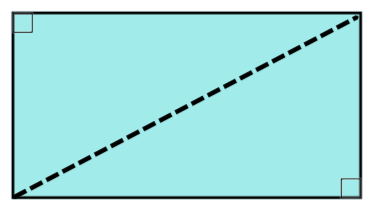Notice that the diagonal of the rectangle connects two opposite corners. It also creates two congruent triangles. Congruent means of equal measure, so the two triangles are the same size, and take up the same amount of space. We should also point out that the triangles are right triangles, because one of their angles is a 90 degree angle (taken from the 90 degree angles of the rectangle).

Let's take a closer look at the rectangle and the two triangles that the diagonal created. The sides of the rectangle correspond to the vertical and horizontal legs of the right triangle. What about the diagonal? We can refer to the diagonal as the hypotenuse of the right triangle. (The hypotenuse is always opposite of the right angle).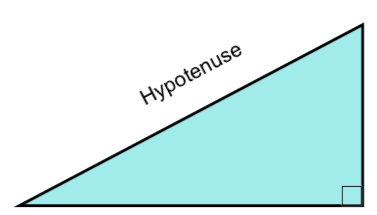# 2. The Pythagorean Theorem

To calculate the length of the diagonal, we can use the Pythagorean Theorem to calculate the length of the hypotenuse. The Pythagorean Theorem uses the side lengths of the other legs of the right triangle in order to find the length of the hypotenuse:

formula
The Pythagorean Theorem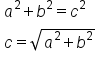So we if take the sum of the squares of the side lengths, this equals the square of the hypotenuse leg. We'll just need to take the square root of the sum in order to express the length of the hypotenuse. Let's look at an example: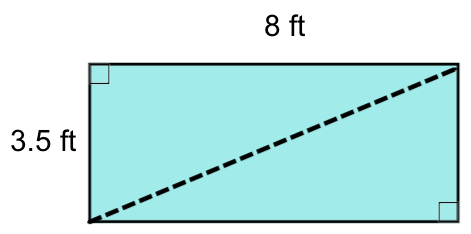We can substitute 3.5 ft and 8 ft into a and b, respectively, and apply the Pythagorean Theorem: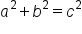The Pythagorean Theorem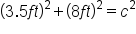Substituting the measurements of the leg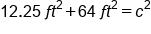Square 3.5 ft and 8 ft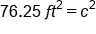Add 12.25 and 64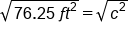Apply the square root of both sides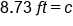Our Solution, rounded to the tenth place

Another method to solving is to rewrite the Pythagorean Theorem, isolating c on one side of the equation. Then, we can substitute a and b into the equation, and calculate the length of the diagonal. This is shown in the example below:The pythagorean Theorem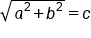Take the square root of both sides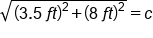Substitute a and b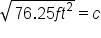Square 3.5 and 8, then add togetherOur Solution, rounded to the tenth place

summary
The diagonal of a rectangle or square corresponds to the hypotenuse of a right triangle. To calculate the diagonal, we can use the Pythagorean Theorem, which we use for right triangles, to calculate the diagonal of a rectangle or square. There are two different forms of the Pythagorean Theorem. Remember that c in our formula has to be the hypotenuse, but a and b can be either of the legs of our right triangle.

Formulas to Know
Pythagorean TheoremRating